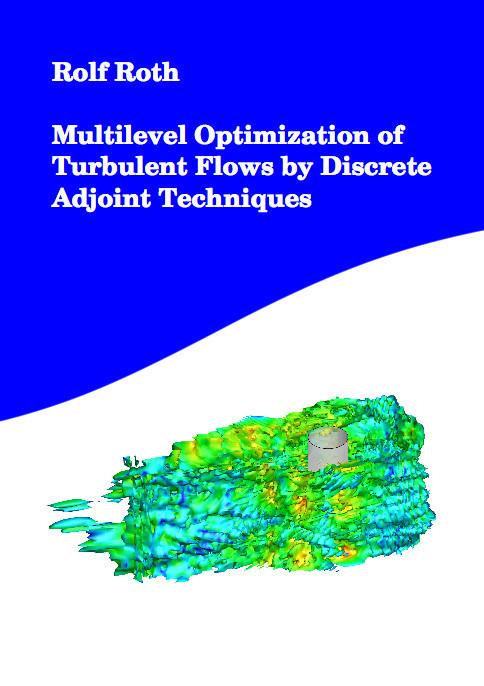Datenbestand vom 20. August 2019aktualisiert am 20. August 2019

# ISBN 9783843905527Euro 84,00 inkl. 7% MwSt

978-3-8439-0552-7, Reihe Mathematik

Rolf Roth
Multilevel Optimization of Turbulent Flows by Discrete Adjoint Techniques

232 Seiten, Dissertation Technische Universität Darmstadt (2012), Softcover, B5

## Zusammenfassung / Abstract

Flow control is an important field of research in mathematics as well as engineering sciences. Major examples are the shape optimization of airfoils, the control of turbulent boundary layers by suction and blowing or the electromagnetic control of conductive fluids.

The mathematical description of fluid flows is given by a coupled system of partial differential equations (PDE). Most of the models used by engineers in practice lack satisfying mathematical theory. Nevertheless, an exception is the Smagorinsky turbulence model, which is used by practitioners and theorists. We present optimal control theory for a class of non-Newtonian fluids, which include this model. These results give motivation to the numerical optimization of turbulent fluid flows.

Afterwards, we consider the efficient numerical treatment of PDE-constrained optimization problems. The ever-increasing speed of modern computers and appropriate numerical algorithms allow not only for the simulation, but also for the optimization of complex problems. In recent years, multilevel optimization algorithms have gained much attention. These optimization algorithms make use of a hierarchical order of models describing a problem. Examples are discretization levels or models with varying physical fidelity. Some further developments in this field of research will be presented.

Many efficient optimization algorithms work with gradient information. Therefore, we developed a discrete adjoint approach that is able to provide gradient information from sophisticated simulation codes. The method makes use of the existing structure of the underlying problem and solver. In particular, we address sparsity exploitation, parallelization and multigrid techniques.

The results and developments were applied to a complex flow solver and various optimization problems governed by fluid flows will be presented. The numerical results include three-dimensional, instationary and turbulent flows.